## STAM101 :: Lecture 19 :: 2Square Factorial Experiments in RBD – lay out – analysis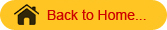2Sqaure Factorial Experiments in RBD
22  factorial experiment means two factors each at two levels. Suppose the two factors are A and B and both are tried with two levels the total number of treatment combinations will be four i.e. a0b0, a0b1, a1b0 and a1b1.
The allotment of these four treatment combinations will be as allotted in RBD. That is each block is divided into four experimental units. By using the random numbers these four combinations are allotted at random for each block separately.
The analysis of variance table for two factors A with a levels and B with b levels with r replications tried in RBD will be as follows:

 Sources of Variation d.f. SS MS F Replications r-1 RSS RMS Factor A a-1 ASS AMS AMS / EMS Factor B b-1 BSS BMS BMS / EMS AB (interaction) (a-1)(b-1) ABSS ABMS ABMS / EMS Error (r-1)(ab-1) ESS EMS Total rab-1 TSS

As in the previous designs calculate the replication totals to calculate the RSS, TSS in the usual way. To calculate ASS, BSS and ABSS, form a two way table A X B by taking the levels of A in rows and levels of B in the columns.  To get the values in this table the missing factor is replication. That is by adding over replication we can form this table.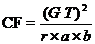RSS =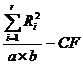A X B Two way table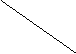B                   A b0 b1 Total a0 a0 b0 a0 b1 A0 a1 a1 b0 a1 b1 A1 Total B0 B1 Grand Total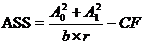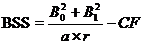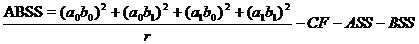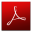Download this lecture as PDF here